# LOD rules

This section contains a detailed description of the different options for entering or selecting the residue values to be used in the food exposure assessments for acute, multi-day and within-day assessment especially as relates to measurement values below the Limit of Detection (LOD) or the Limit of Quantification (LOQ). The outputs of these options will be combined with the food consumption data.

The input residue values below the LOD or the LOQ can be modified according to certain rules that depend on the type of residue data used (monitoring, field trials or tolerance level), on the classification of each RAC as blended, partially blended or not blended and on the fraction of crop treated related to each RAC. In this document, these rules will be called LOD/LOQ rules which are based on the EPA guidelines.

Office of Pesticide Programs U.S. Environmental Protection Agency. (2003). Assigning values to non-detected/non-quantified pesticide residues in human health food exposure assessments. Washington, DC: U.S. Environmental Protection Agency.

These rules modify the non-detected values included in the input residue data in order to match the number of zero values with the fraction of crop treated. These rules are based on the values of the LOD or LOQ provided by the user together with the residue values. CARES NG® will accept either the LODs or the LOQs but it will not make any distinctions between the two sets of values; LODs and LOQs will be treated in the same way. It will be a user’s responsibility to insert the correct set of values into the CARES NG software.

In the cumulative assessments, the LOD/LOQ rules are not applicable because it is necessary to preserve the co-occurrence of the different pesticides in the analysed foods. Therefore the non-detected values will be treated as true zeros and no further changes will be made to the input residue data.

For each food consumption event, CARES NG® randomly selects a residue value from the modified distribution of the residue values. The randomly selected residue value is then multiplied by the processing factor(s).

# Monitoring Data

Generally, these residue data come from pesticide monitoring programs such as the U.S. Pesticide Data Program (PDP) which collects, analyses and reports pesticide residues on agricultural commodities in the U.S. food supply on a yearly basis. The PDP database will be included in the CARES NG software.
CARES NG® gives the user the choice to either modify the input residue values by applying the LOD/LOQ rules or consider the non-detected values as true zeros. The distribution of residue values will be determined according to the following process.

## Partially Blended and Not Blended Commodities

In case the user chooses to apply the LOD/LOQ rules, the software will return a distribution of residue values having the following characteristics:

1. The detected values present in the monitoring database will be all included in the final distribution
2. The proportion of true zeros in the final distribution has to be equal or lower than the fraction of crop treated provided as input. Therefore the number of zeros will be determined according to the following formula:

•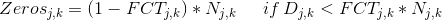•for each pesticide j=1,…,M and for each RAC k=1,…,K. Nj,k, Dj,k and FCTj,k are the size and the number of detected residue value of the monitoring database and the fraction crop treated of the j-th pesticide and of the k-th RAC, respectively. Zerosj,k is the number of zeros to be included in the final distribution of the j-th pesticide and of the k-th RAC.

3. If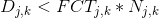, then a number of non-detected values recorded in the residue database will be substituted with the average value of the ½ LOD/LOQs of the methods used to measure the pesticide concentrations of the only non-detected samples; the LOD/LOQs of the detected samples are excluded from the calculation of the average ½ LOD/LOQ. The average value is a weighted average calculating with the number of samples collected using each method. The number of non-detected to be substituted will be:for each pesticide j=1,…,M and for each RAC k=1,…,K. NDj,k is the number of non-detected values to be substituted with the average ½ LOD/LOQ in the final distribution of the j-th pesticide and of the k-th RAC.

The final distribution of the residue values to be used in the Food Model for calculating the acute, multi-day and within-day exposure levels will have the following form: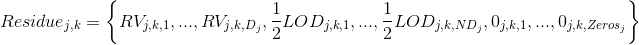for each pesticide j=1,…,M and for each RAC k=1,…,K. RVj,k,n is the n-th residue value reported in the monitoring database for j-th pesticide and for the k-th RAC.

In case the user chooses to consider all the non-detected values as zeros, the CARES NG software will replace all the non-detected values present in the input data with zeros. No further changes will be made to the input data. The fraction of crop treated of the RAC(s) will not have any effect on the data and will not be used in any calculation with this option.

## Blended Commodities

In case the user chooses to apply the LOD/LOQ rules, all the non-detected value of input residue database will be replaced with the average ½ LOD/LOQ value for that RAC, calculated as previously explained and the average residue values will be calculated over the resulting distribution. The average value will be multiplied by the fraction of crop treated FCTj,k for each RAC and for each pesticide (M) under analysis.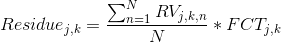Otherwise, the non-detected values will be replaced with zeros and the average residue values will be calculated over the resulting distribution:# Field-Trial Data

These residue data come from user’s analyses and no default databases will be included in the CARES NG software. Typically these analyses are carried out on crops which were treated with the pesticide(s) under analysis. The non-detected values cannot be considered as true zeros; therefore the CARES NG software will not give the user the choice to not apply the LOD/LOQ rules to the field trial data.
The distribution of residue values will be determined according to the following which provides a process to incorporate Fraction Crop Treated values into field trial data used in acute, multi-day, and within-day assessments.

## Partially Blended and Not Blended Commodities

CARES NG® returns a distribution of residue values having the following characteristics:

1. The detected values present in the input residue database are all included in the final distribution
2. The size of the final distribution Nj,k is equal to: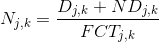for each pesticide j=1,…,M and for each RAC k=1,…,K
3. The number of true zeros in the distribution is equal to:for each pesticide j=1,…,M and for each RAC k=1,…,K. Nj,k, NDj,k and Dj,k are the size of the final distribution, the number of non-detected and the number of detected residue value of the j-th pesticide and of the k-th RAC, respectively. Zerosj,k is the number of zeros to be included in the final distribution of the j-th pesticide and of the k-th RAC.
4. The non-detected values recorded in the residue database are substituted with the average value of the ½ LOD/LOQs of the methods used to measure the pesticide concentrations of only the non-detected samples; the LOD/LOQs of the detected samples are excluded from the calculation of the average ½ LOD/LOQ. The average value is calculated weighted by the number of samples collected using each method.

The final distribution of the residue values to be used in the Food Model for calculating the acute, multi-day and within-day exposure levels will have the following form:for each pesticide j=1,…,M and for each RAC k=1,…,KRVj,k,n is the n-th residue value reported in the monitoring database for j-th pesticide and for the k-th RAC.

## Blended Commodities

All the non-detected value of input residue database will be replaced with the average ½ LOD/LOQ value, calculated as previously explained, and the average residue values will be calculated over the resulting distribution. The average value will be multiplied by the percentage of crop treated FCTj,k for each of the M pesticides under analysis.# Tolerance Values

For the blended commodities, the tolerance level is multiplied times the percentage of crop treated of the commodity under analysis. The residue value to be used in the Food Model is equal to:for each pesticide j=1,…,M and each RAC k=1,…,K

For the partially-blended and not-blended commodities, the CARES NG software will add to the tolerance level a number of zeros values based on the FCTk of the k-th RAC under analysis, as per the following formula:for each pesticide j=1,…,M and for each RAC k=1,…,K

The final distribution of the residue values to be used in the Food Model for calculating the exposure levels will have the following form:for each pesticide j=1,…,M and for each RAC k=1,…,K